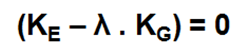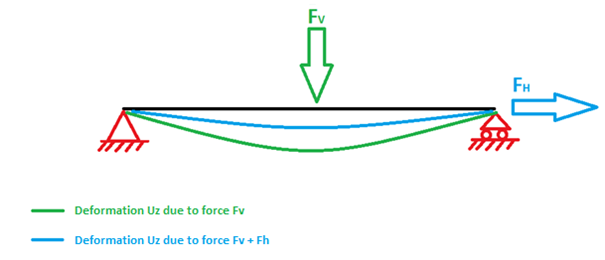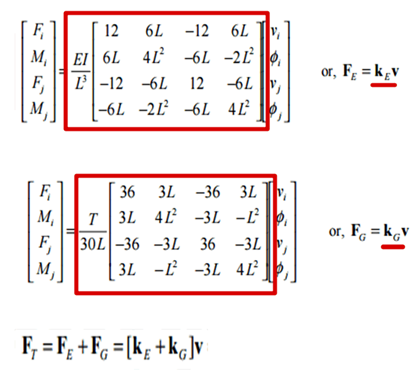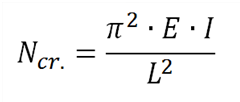# How is the critical load coefficient determined via a stability calculation?

The critical load coefficient is determined by solving an eigenvalue problem via the following equation:Ke = Elastic stiffness matrix EI
Kg = Geometrical stiffness matrix of the normal forces T
Lambda = eigenvalue = critical load coefficient

The elastic stiffness matrix is known because it represents the stiffness of the structure elements (columns, beams,…).
The geometrical stiffness matrix is a correction matrix to get a more realistic stiffness of the structure. I will explain the purpose of the geometrical stiffness matrix with a simple example. Consider a beam on two supports which is loaded as follows:The beam will deform according to the green line if it is only loaded by the vertical load Fv. The value of the deformation is related to the elastic stiffness (Stiffness matrix Ke) of that element.
This beam will deform less (blue line) when there is besides the vertical load Fv a horizontal load Fh. This horizontal load Fh has a stiffening effect on the vertical deformation of the beam. This stiffening effect is taken into account in the geometrical stiffness matrix Kg. Kg is in fact a correction on Ke in order to get realistic results of the structure. SCIA Engineer searches during the stability calculation for a lambda value that fulfills the following equation
Ke = Lambda x Kg.
This is done by solving the stiffness matrices of the above equation. Below you can find an example of such stiffness matrices (Ke & Kg).Extra information:
Once the critical load coefficient is determined via the stability calculation the user can determine the critical normal force Ncr. that causes instability (=buckling) of the structure. Because Ncr. = alpha_critical x Ned (Formula 5.1 of EN1993-1-1; Lambda = alpha_critical).

The user can also determine the buckling factors ky & kz when he knows Ncr. Because the buckling factors are the only unknowns in the formula of Euler:(with L=bucklinglength = Ky x systemlength yy ; kz x systemlenght zz)